# Alternating group:A4

(Redirected from SmallGroup(12,3))
View a complete list of particular groups (this is a very huge list!)[SHOW MORE]

## Definition

The alternating group$A_4$ is defined in the following equivalent ways:

• It is the group of even permutations (viz., the alternating group) on four elements.
• It is the von Dyck group (sometimes termed triangle group, though triangle group has an alternative interpretation) with parameters$(2,3,3)$ (sometimes written in reverse order as$(3,3,2)$).
• It is the group of orientation-preserving symmetries of a regular tetrahedron. When viewed in this light, it is called the tetrahedral group, and its symbol as a point group is$T$ or$332$. Further information: Classification of finite subgroups of SO(3,R), Linear representation theory of alternating group:A4
• It is the projective special linear group of degree two over the field of three elements, viz.,$PSL(2,3)$.
• It is the general affine group of degree$1$ over the field of four elements, viz.,$GA(1,4)$ (also written as$AGL(1,4)$.

### Equivalence of definitions

IMPORTANT NOTE: This page concentrates on$A_4$ as an abstract group in its own right. To learn more about this group as a subgroup of index two inside symmetric group:S4, see A4 in S4.

## Arithmetic functions

Want to compare and contrast arithmetic function values with other groups of the same order? Check out groups of order 12#Arithmetic functions

### Basic arithmetic functions

Function Value Similar groups Explanation
order (number of elements, equivalently, cardinality or size of underlying set) 12 groups with same order As$A_n, n = 4$:$n!/2 = 4!/2 = 12$
As$PSL(2,q), q = 3$:$(q^3 - q)/\operatorname{gcd}(2,q - 1) = (3^3 - 3)/2 = 12$
As$GA(1,q), q = 4$:$q(q - 1) = 4(3) = 12$
As von Dyck group with parameters$(p,q,r) = (2,3,3)$:$\frac{2}{1/p + 1/q + 1/r - 1} = \frac{2}{1/2 + 1/3 + 1/3 - 1} = \frac{2}{1/6} = 12$
exponent of a group 6 groups with same order and exponent of a group | groups with same exponent of a group Elements of order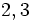$2,3$.
derived length 2 groups with same order and derived length | groups with same derived length Derived series goes through Klein four-group of double transpositions.
nilpotency class -- -- not a nilpotent group.
Frattini length 1 groups with same order and Frattini length | groups with same Frattini length Frattini-free group: intersection of maximal subgroups is trivial.
minimum size of generating set 2 groups with same order and minimum size of generating set | groups with same minimum size of generating set$(1,2,3), (1,2)(3,4)$
subgroup rank of a group 2 groups with same order and subgroup rank of a group | groups with same subgroup rank of a group
max-length of a group 3 groups with same order and max-length of a group | groups with same max-length of a group
composition length 3 groups with same order and composition length | groups with same composition length
chief length 2 groups with same order and chief length | groups with same chief length The unique minimal normal subgroup is also the unique maximal normal subgroup and has order$2^2 = 4$ and index$3$.

### Arithmetic functions of a counting nature

Function Value Similar groups Explanation
number of conjugacy classes 4 groups with same order and number of conjugacy classes | groups with same number of conjugacy classes As$A_n, n = 4$: (Number of pairs of non-self-conjugate partitions of$n$) + 2(Number of self-conjugate partitions of$n$) = 2 + 2(1) = 4
As$PSL(2,q), q = 3$:$(q + 5)/2 = (3 + 5)/2 = 4$
As$GA(1,q), q = 4$,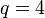$q = 4$
See element structure of alternating group:A4#Number of conjugacy classes for more information.
number of orbits under automorphism group 3 groups with same order and number of orbits under automorphism group | groups with same number of orbits under automorphism group See element structure of alternating group:A4. The two conjugacy classes of elements of order three are fused under the action of the automorphism group.
number of equivalence classes under real conjugacy 3 groups with same order and number of equivalence classes under real conjugacy | groups with same number of equivalence classes under real conjugacy See element structure of alternating group:A4. The two conjugacy classes of elements of order three are fused under real conjugacy because they are inverses of each other.
number of equivalence classes under rational conjugacy 3 groups with same order and number of equivalence classes under rational conjugacy | groups with same number of equivalence classes under rational conjugacy See element structure of alternating group:A4. The two conjugacy classes of elements of order three are fused under the action of the automorphism group.
number of conjugacy classes of real elements 2 groups with same order and number of conjugacy classes of real elements | groups with same number of conjugacy classes of real elements See element structure of alternating group:A4. The identity element and the conjugacy class of double transpositions are the only such classes.
number of conjugacy classes of rational elements 2 groups with same order and number of conjugacy classes of rational elements | groups with same number of conjugacy classes of rational elements See element structure of alternating group:A4. The identity element and the conjugacy class of double transpositions are the only such classes.
number of conjugacy classes of subgroups 5 groups with same order and number of conjugacy classes of subgroups | groups with same number of conjugacy classes of subgroups See subgroup structure of alternating group:A4
number of subgroups 10 groups with same order and number of subgroups | groups with same number of subgroups See subgroup structure of alternating group:A4

## Group properties

Property Satisfied? Explanation Comment
Abelian group No$(1,2,3)$,$(1,2)(3,4)$ don't commute$A_n$ is non-abelian,$n \ge 4$.
Nilpotent group No Centerless: The center is trivial$A_n$ is non-nilpotent,$n \ge 4$.
Metacyclic group No No cyclic normal subgroup$S_n$ is not metacyclic,$n \ge 4$.
Supersolvable group No No cyclic normal subgroup$S_n$ is not supersolvable,$n \ge 4$.
Solvable group Yes Length two, derived subgroup is Klein four-group Largest$n$ for which$A_n$ is solvable.
T-group No Double transposition generates non-normal 2-subnormal subgroup Only$n$ for which$A_n$ isn't a T-group.
Group having subgroups of all orders dividing the group order No No subgroup of order six
Ambivalent group No Classification of ambivalent alternating groups
Rational group No
Rational-representation group No
Group in which every element is automorphic to its inverse Yes Alternating group implies every element is automorphic to its inverse
Group in which any two elements generating the same cyclic subgroup are automorphic Yes Alternating group implies any two elements generating the same cyclic subgroup are automorphic
Frobenius group Yes Frobenius kernel is Klein four-subgroup, complement is generated by 3-cycle
Camina group Yes Derived subgroup is Klein four-subgroup, other two cosets are conjugacy classes.

## Endomorphisms

### Automorphisms

The automorphism group of the alternating group of degree four is isomorphic to the symmetric group of degree four. Since the alternating group of degree four is centerless, it embeds as a subgroup inside its automorphism group.

Another way of thinking of this is as follows: in the symmetric group of degree four, the alternating group of degree four is a subgroup of index two, and every automorphism of this subgroup is realized as the restriction to this subgroup of an inner automorphism of the symmetric group.

### Endomorphisms

The endomorphisms of the alternating group of degree four are given by the following (i.e., equivalent to one of these up to composition with an automorphism):

• The trivial map.
• The identity map.
• The retraction to a subgroup of order three, with kernel being the Klein four-group comprising the identity and the double transpositions. (All such retractions are equivalent).

## Elements

Further information: element structure of alternating group:A4

### Summary

Item Value
order of the whole group (total number of elements) 12 (see order computation for more)
conjugacy class sizes 1,3,4,4
maximum: 4, number: 4, sum (equals order of whole group): 12, lcm: 12
See conjugacy class structure for more.
number of conjugacy classes 4
See number of conjugacy classes for more.
order statistics 1 of order 1, 3 of order 2, 8 of order 3
maximum: 3, lcm (exponent of the whole group): 6

### Up to conjugacy

For a symmetric group, cycle type determines conjugacy class. The statement is almost true for the alternating group, except for the fact that some conjugacy classes of even permutations in the symmetric group split into two in the alternating group, as per the splitting criterion for conjugacy classes in the alternating group, which says that a conjugacy class of even permutations splits in the alternating group if and only if its cycle decomposition comprises odd cycles of distinct length.

Here are the unsplit conjugacy classes:

Partition Verbal description of cycle type Elements with the cycle type Size of conjugacy class Formula for size Element order
1 + 1 + 1 + 1 four cycles of size one each, i.e., four fixed points$()$ -- the identity element 1$\! \frac{4!}{(1)^4(4!)}$ 1
2 + 2 double transposition: two cycles of size two$(1,2)(3,4)$,$(1,3)(2,4)$,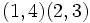$(1,4)(2,3)$ 3$\! \frac{4!}{(2)^2(2!)}$ 2
Total --$()$,$(1,2)(3,4)$,$(1,3)(2,4)$ and$(1,4)(2,3)$ 4 NA NA

In this case, the union of the unsplit conjugacy classes is a proper normal subgroup isomorphic to the Klein four-group. Note that this phenomenon is unique to the case$n = 4$.

Here is the split conjugacy class:

Partition Verbal description of cycle type Elements with the cycle type Combined size of conjugacy classes Formula for combined size Size of each half First split half Second split half Real? Rational? Element order
3 + 1 one 3-cycle, one fixed point$(1,2,3)$,$(1,3,2)$,$(2,3,4)$,$(2,4,3)$,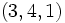$(3,4,1)$,$(3,1,4)$,$(4,1,2)$,$(4,2,1)$ 8$\! \frac{4!}{(3)(1)}$ 4$(1,2,3)$,$(4,2,1)$,$(2,4,3)$,$(3,4,1)$$(1,3,2)$,$(4,1,2)$,$(2,3,4)$,$(3,1,4)$ No No 3

### Up to automorphism

The conjugacy classes of the identity element and double transpositions are invariant under all automorphisms.

An outer automorphism interchanges the conjugacy classes of elements of order three (each of size four). This can be realized, for instance, by viewing the alternating group as a subgroup of the symmetric group of degree four. Any transposition or$4$-cycle in the symmetric group interchanges those two conjugacy classes

## Subgroups

Further information: Subgroup structure of alternating group:A4

### Quick summary

Item Value
Number of subgroups 10
Number of conjugacy classes of subgroups 5
Number of automorphism classes of subgroups 5

### Table classifying subgroups up to automorphism

Automorphism class of subgroups List of subgroups Isomorphism class Order of subgroups Index of subgroups Number of conjugacy classes Size of each conjugacy class Total number of subgroups Isomorphism class of quotient (if exists) Subnormal depth (if subnormal) Note
trivial subgroup$\{ () \}$ trivial group 1 12 1 1 1 alternating group:A4 1 trivial
subgroup generated by double transposition in A4$\{ (), (1,2)(3,4) \}$,$\{ (), (1,3)(2,4) \}$,$\{ (), (1,4)(2,3) \}$ cyclic group:Z2 2 6 1 3 3 -- 2
V4 in A4$\{ (), (1,2)(3,4), (1,3)(2,4), (1,4)(2,3) \}$ Klein four-group 4 3 1 1 1 cyclic group:Z3 1 2-Sylow, minimal normal, maximal
A3 in A4$\{ (), (2,3,4), (2,4,3) \}$,$\{ (), (1,3,4), (1,4,3)\}$,$\{ (), (1,2,4), (1,4,2)\}$,$\{ (), (1,2,3), (1,3,2) \}$ cyclic group:Z3 3 4 1 4 4 -- -- 3-Sylow, maximal
whole group all elements alternating group:A4 12 1 1 1 1 trivial group 1 whole
Total (5 rows) -- -- -- -- 5 -- 10 -- -- --

## Supergroups

Further information: supergroups of alternating group:A4

### Subgroups: making all the automorphisms inner

Further information: symmetric group:S4, A4 in S4

The outer automorphism group of alternating group:A4 is cyclic group:Z2 and the automorphism group is symmetric group:S4. Since$A_4$ is centerless, it equals its inner automorphism group and hence embeds as a subgroup of index two inside symmetric group:S4.

In particular, symmetric group:S4 is the unique group containing alternating group:A4 as a NSCFN-subgroup (a normal fully normalized subgroup that is also a self-centralizing subgroup).

### Quotients: Schur covering groups

Further information: special linear group:SL(2,3), center of special linear group:SL(2,3)

The Schur multiplier of alternating group:A4 is cyclic group:Z2. There is a unique corresponding Schur covering group, namely the group special linear group:SL(2,3), where the center of special linear group:SL(2,3) is isomorphic to the Schur multiplier cyclic group:Z2 and the quotient is alternating group:A4.

The Schur covering group$SL(2,3)$ is also denoted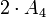$2 \cdot A_4$ to indicate that it is a double cover of alternating group.

## Endomorphisms

Further information: endomorphism structure of alternating group:A4

Construct Value Order Second part of GAP ID (if group)
endomorphism monoid  ? 33 --
automorphism group symmetric group:S4 24 12
inner automorphism group alternating group:A4 12 3
outer automorphism group cyclic group:Z2 2 1
extended automorphism group direct product of S4 and Z2 48 48

## Extensions

These are groups having the alternating group as a quotient group Perhaps the most important of these is$SL(2,3)$, which is the universal central extension of$PSL(2,3)$. The kernel of the projection mapping is a two-element subgroup, namely the identity matrix and the negative identity matrix.

## GAP implementation

### Group ID

This finite group has order 12 and has ID 3 among the groups of order 12 in GAP's SmallGroup library. For context, there are 5 groups of order 12. It can thus be defined using GAP's SmallGroup function as:

SmallGroup(12,3)

For instance, we can use the following assignment in GAP to create the group and name it$G$:

gap> G := SmallGroup(12,3);

Conversely, to check whether a given group$G$ is in fact the group we want, we can use GAP's IdGroup function:

IdGroup(G) = [12,3]

or just do:

IdGroup(G)

to have GAP output the group ID, that we can then compare to what we want.

### Other definitions

The alternating group can be constructed in many equivalent ways:

• As the alternating group of degree four, using GAP's AlternatingGroup function:

AlternatingGroup(4)

• Using the von Dyck presentation. Here is a sequence of steps:
F := FreeGroup(3);
G := F/[F.1^3, F.2^3, F.3^2, F.1*F.2*F.3]

The output$G$ is the alternating group.

PSL(2,3)